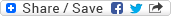## Friday, May 23, 2008

### Adobe - Reverse the order of words in a char seq

Question: Write an efficient algorithm to Reverse the order of words in a given array of character which form a sequence of words.

Note: Memory-based question, suppodely a part of 'Engineering' section of the written test for a Dev position at Adobe.

Solution: Find below one possible algorithm to solve this problem:-

• Allocate memory for another array of same size
• Iterate through the array and find words (by finding white spaces)
• Put the word reversed (order of characters reversed) in the new array
• Put the white spaces as well (you won't reverse them as they are same either way)
• Continue and complete the iteration for all the words in the array
• Copy the new array reversed into the original array and you're done!

Example: Suppose you have one array "int char float" in the beginning. The expected result should be "float char int" in this case. Let's dry run the above algorithm for this test array and see if it gets us the expected result.

NewArray = " " (a array of 14 characters, currently all blank)

Iteration starts here

First word found: "int", so NewArray = "tni "

Second word found: "char", so NewArray = "tni rahc "

Third word found: "float", so NewArray = "tni rahc taolf"

Iteration ends here

Now reverse of the NewArray = "float char int"Anonymous said...

I had this question. The questioner said the goal was not to reverse the order of the letters in the words, but instead, to reverse the order of the words (like it says).

Ex: "The fat cat"
becomes "cat fat The"

Author:Rahul Chintakunta
Email:c.rahulkumar@gmail.com

The below program serves the purpose:

class RevCharArr1 {

public static void main(String[] args) {

String input = "HOW ARE YOU?";
String expectedOut = "YOU? ARE HOW";

char data[] = input.toCharArray();
char temp[] = new char[data.length];

int count = 0;
int start = 0;
int end = 0;

char[] finalArr = new char[data.length];

for(int i=data.length-1;i>=0;i--){
if(data[i] == ' '){
reverse(finalArr, temp, start, end);
start = end + 1;
}
temp[count++] = data[i];
end++;
}
reverse(finalArr, temp, start, end);
System.out.println(temp);
System.out.println(finalArr);
}

public static void reverse(char[] finalArr, char[] temp, int start, int end){
int count = end - start;
int tempCount = 0;
while(count != tempCount){
finalArr[start++] = temp[--end];
tempCount++;
}
if(start != finalArr.length)
finalArr[start++] = ' ';
}
}

public class RevCharArr {

public static void main(String[] args) {

String input = "The fat cat";

char data[] = input.toCharArray();

char temp[] = new char[data.length];

int count = 0;

int start = 0;

int end = 0;

char[] finalArr = new char[data.length];

for(int i=data.length-1;i>=0;i--){

if(data[i] == ' '){

reverse(finalArr, temp, start, end);

start = end + 1;

}

temp[count++] = data[i];

end++;

}

reverse(finalArr, temp, start, end);

System.out.println(finalArr);

}

public static void reverse(char[] finalArr, char[] temp, int start, int end){

int count = end - start;

int tempCount = 0;

while(count != tempCount){

finalArr[start++] = temp[--end];

tempCount++;

}

if(start != finalArr.length)

finalArr[start++] = ' ';

}

}